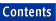Apache C++ Standard Library Reference Guide

## nth_element()

Library:  Algorithms

`Function`

No Entries

### Summary

An algorithm that rearranges a collection so that all elements lower in sorted order than the nth element come before it, and all elements higher in sorted order than the nth element come after it

### Synopsis

```#include <algorithm>

namespace std {
template <class RandomAccessIterator>
void nth_element(RandomAccessIterator start,
RandomAccessIterator nth,
RandomAccessIterator finish);

template <class RandomAccessIterator, class Compare>
void nth_element(RandomAccessIterator start,
RandomAccessIterator nth,
RandomAccessIterator finish,
Compare comp);
}
```

### Description

The nth_element() algorithm rearranges a collection according to either operator<() or the function object comp. After the algorithm is applied, the follwoing hold:

• The element that would be in the nth position if the sequence were completely sorted is in the nth position

• All elements prior to the nth position would also precede that position in an ordered sequence

• All elements following the nth position would also follow that position in an ordered sequence

That is, for any iterator i in the range [start, nth) and any iterator j in the range [nth, finish), it holds that !(*i > *j) or comp(*i, *j) == false.

Note that the elements that precede or follow the nth position are not necessarily sorted relative to each other. The nth_element() algorithm does not sort the entire collection.

### Complexity

The algorithm is linear, on average, where N is the size of the range [start, finish).

### Example

```//
//  nthelem.cpp
//

#include <algorithm>   // for swap
#include <vector>      // for vector
#include <iostream>    // for cout, endl

template<class RandomAccessIterator>
void quik_sort (RandomAccessIterator start, RandomAccessIterator end)
{
size_t dist = end - start;

// Stop condition for recursion.
if (dist > 2) {
// Use nth_element to do all the work for quik_sort.
std::nth_element (start, start + (dist / 2), end);

// Recursive calls to each remaining unsorted portion.
quik_sort (start, start + (dist / 2 - 1));
quik_sort (start + (dist / 2 + 1), end);
}

if (2 == dist && *(end - 1) < *start)
std::swap (start, end);
}

int main ()
{
typedef std::vector<int, std::allocator<int> > Vector;

// Initialize a vector using an array of integers.
Vector::value_type arr[] = { 37, 12,  2, -5, 14,
1,  0, -1, 14, 32 };

Vector v (arr + 0, arr + sizeof arr / sizeof *arr);

// Print the initial vector.
std::cout << "The unsorted values are: \n     ";

for (Vector::iterator i = v.begin (); i != v.end (); ++i)
std::cout << *i << ", ";

std::cout << "\n\n";

// Use the new sort algorithm.
quik_sort (v.begin (), v.end ());

// Output the sorted vector.
std::cout << "The sorted values are: \n     ";
for (Vector::iterator i = v.begin (); i != v.end (); ++i)
std::cout << *i << ", ";

std::cout << std::endl << std::endl;

return 0;
}

Program Output:
The unsorted values are:
37, 12, 2, -5, 14, 1, 0, -1, 14, 32,

The sorted values are:
-5, -1, 0, 1, 2, 12, 14, 14, 32, 37,
```